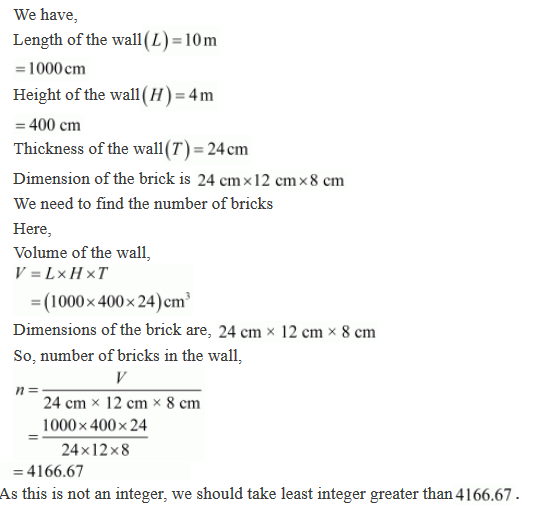#### Chapter 18 Surface Area and Volume of a Cuboid and Cube R.D. Sharma Solutions for Class 9th Exercise 18.2

Exercise 18.2

1
A cuboidal water tank is 6 m long, 5 m wide and 4.5 m deep. How many litres of water can it hold ?

Solution2. A cubical vessel is 10 m long and 8 m wide. How high must it be made to hold 380 cubic metres of a liquid ?

Solution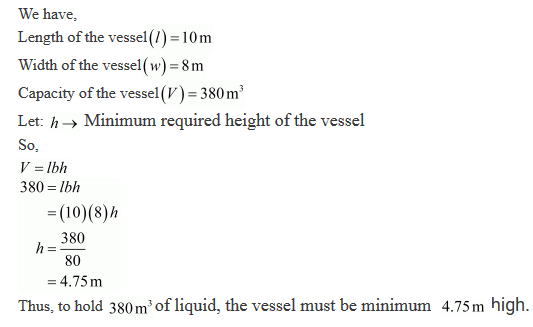3. Find the cost of digging a cuboidal pit 8 m long, 6 m broad and 3 m deep at the rate of Rs 30 per m3.

Solution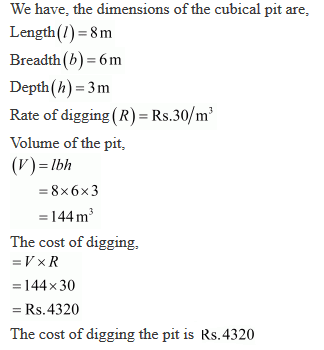4. If V is the volume of a cuboid of dimensions a, b, c and S is its surface area, then prove that
1/V = 2/S (1/a + 1/b + 1/c)

Solution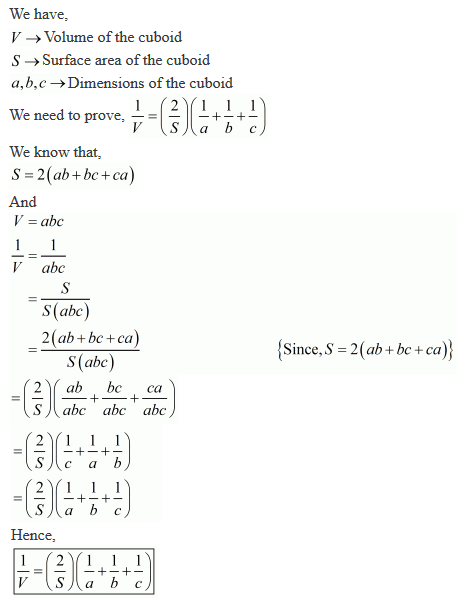5. The areas of three adjacent faces of a cuboid are x, y and z. If the volume is V, prove that V2 = xyz.

Solution6. If the areas of three adjacent faces of a cuboid are 8 cm2, 18 cm3 and 25 cm3. Find the volume of the cuboid.

Solution7. The breadth of a room is twice its height, one half of its length and the volume of the room is 512 cu. dm. Find its dimensions.

Solution8. A river 3 m deep and 40 m wide is flowing at the rate of 2 km per hour. How much water will fall into the sea in a minute ?

Solution9. Water in a canal 30 dm wide and 12 dm deep, is flowing with a velocity of 100 km per hour. How much area will it irrigate in 30 minutes if 8 cm of standing water is desired?

Solution10. Three metal cubes with edges 6 cm, 8 cm and 10 cm respectively are melted together and formed into a single cube. Find the volume, surface area and diagonal of the new cube.

Solution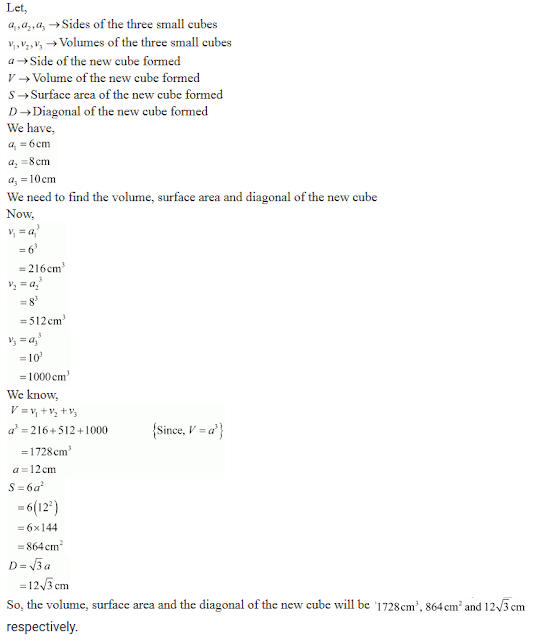11. Two cubes, each of volume 512 cm3 are joined end to end. Find the surface area of the resulting cuboid.

Solution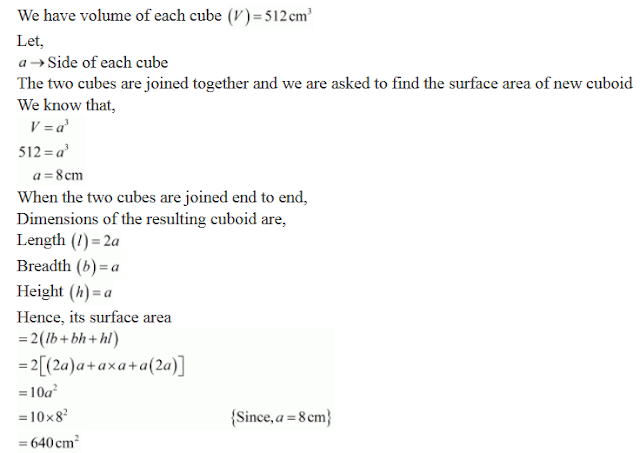12. Half cubic metre of gold-sheet is extended by hammering so as to cover an area of 1 hectare. Find the thickness of the gold-sheet.

Solution13. A metal cube of edge 12 cm is melted and formed into three smaller cubes. If the edge of the two smaller cubes are 6 cm and 8 cm, find the edge of the third smaller cube.

Solution14. The dimensions of a cinema hall are 100 m, 50 m and 18 m. How many persons can sit in the hall, if each person requires 150 m3 of air?

Solution15. Given that 1 cubic cm of marble weighs 0.25 kg, the weight of marble block 28 cm in with and 5 cm thick is 112 kg. Find the length of the block.

Solution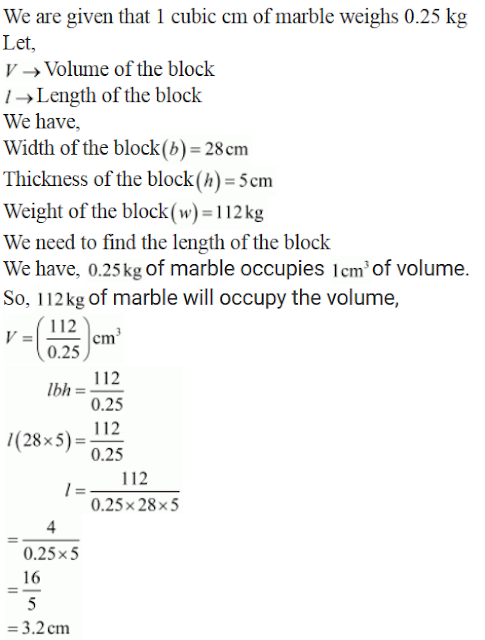16. A box with lid is made of 2 cm thick wood. Its external length, breadth and height are 25 cm, 18 cm and 15 cm respectively. How much cubic cm of a liquid can be placed in it? Also, find the volume of the wood used in it.

Solution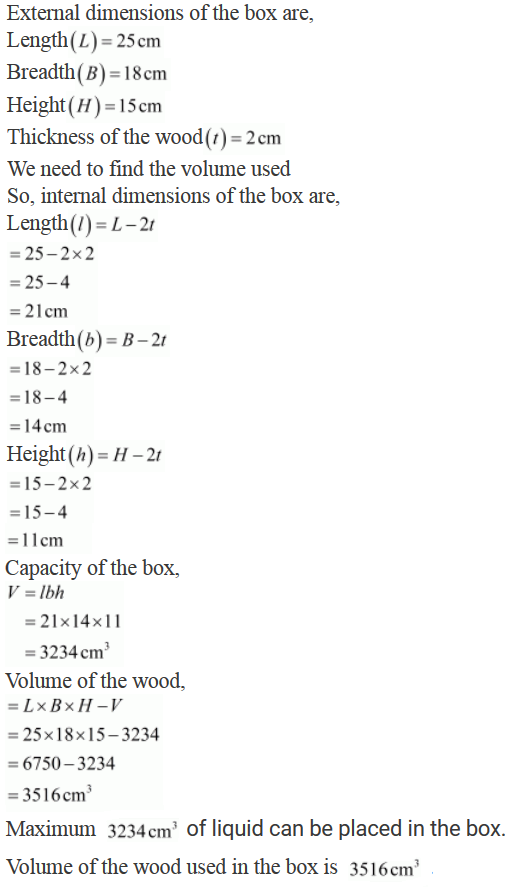17. The external dimensions of a closed wooden box are 48 cm, 36 cm, 30 cm. The box is made of 1.5 cm thick wood. How many bricks of size 6 cm × 3 cm × 0.75 cm can be put in this box ?

Solution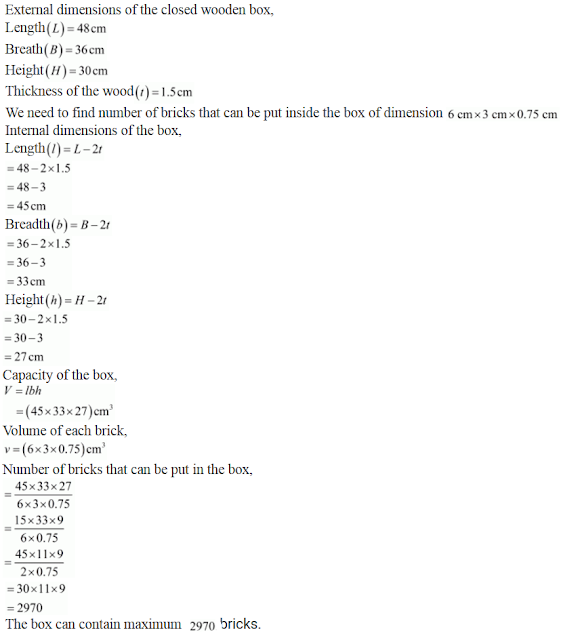18. How many cubic centimetres of iron are there in an open box whose external dimensions are 36 cm, 25 cm and 16.5 cm, the iron being 1.5 cm thick throughout? If 1 cubic cm of iron weighs 15 g, find the weight of the empty box in kg.

Solution19. A cube of 9 cm edge is immersed completely in a rectangular vessel containing water. If the dimensions of the base are 15 cm and 12 cm, find the rise in water level in the vessel.

Solution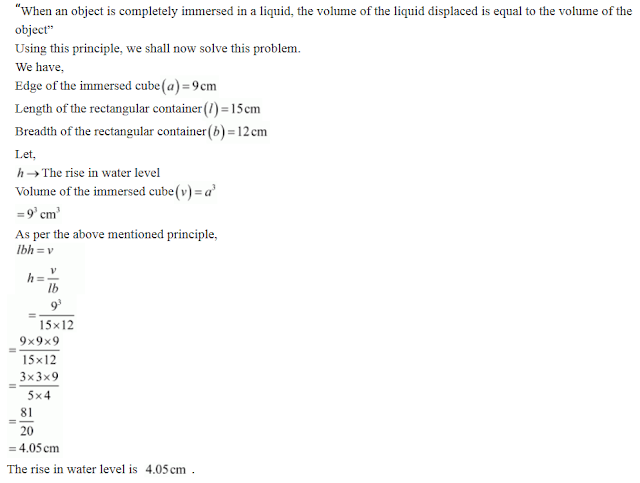20. A rectangular container, whose base is a square of side 5 cm, stands on a horizontal table, and holds water upto 1 cm from the top. When a cube is placed in the water it is completely submerged, the water rises to the top and 2 cubic cm of water overflows. Calculate the volume of the cube and also the length of its edge.

Solution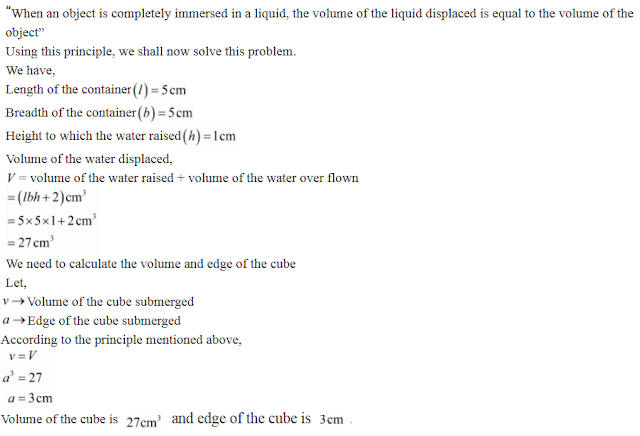21. A field is 200 m long and 150 m broad. There is a plot, 50 m long and 40 m broad, near the field. The plot is dug 7 m deep and the earth taken out is spread evenly on the field. By how many metres is the level of the field raised? Give the answer to the second place of decimal.

Solution22. A field is in the form of a rectangle of length 18 m and width 15 m. A pit, 7.5 m long, 6 m broad and 0.8 m deep, is dug in a corner of the field and the earth taken out is spread over the remaining area of the field. Find out the extent to which the level of the field has been raised.

Solution23. A rectangular tank is 80 m long and 25 m broad. Water flows into it through a pipe whose cross-section is 25 cm2, at the rate of 16 km per hour. How much the level of the water rises in the tank in 45 minutes.

.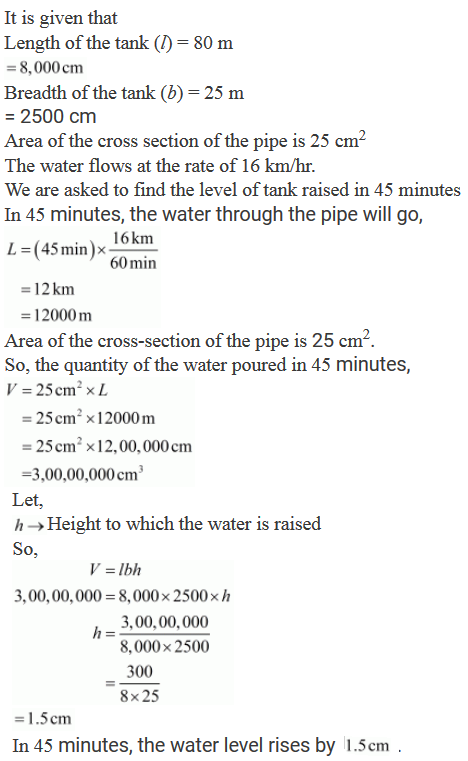24. Water in a rectangular reservoir having base 80 m by 60 m is 6.5 deep. In what time can the water be emptied by a pipe of which the cross-section is a square of side 20 cm, if the water runs through the pipe at the rate of 15 km/hr.

Solution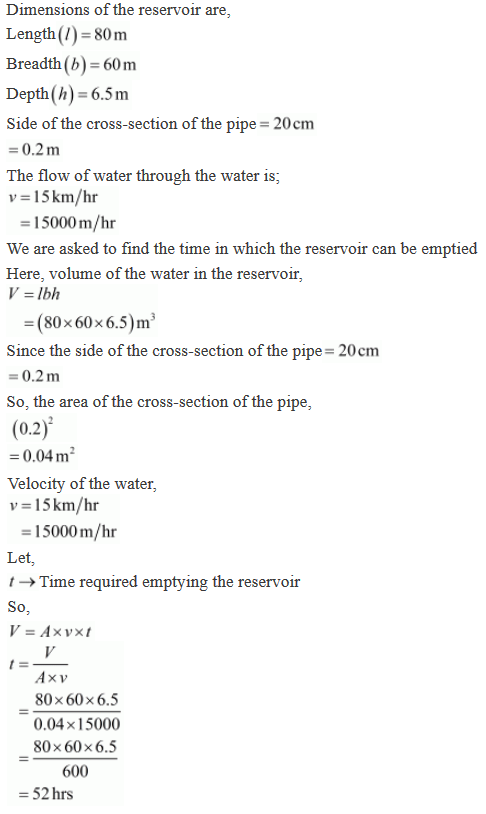25. A village having a population of 4000 requires 150 litres of water per head per day. It has a tank measuring 20 m × 15 m × 6 m. For how many days will the water of this tank last ?

Solution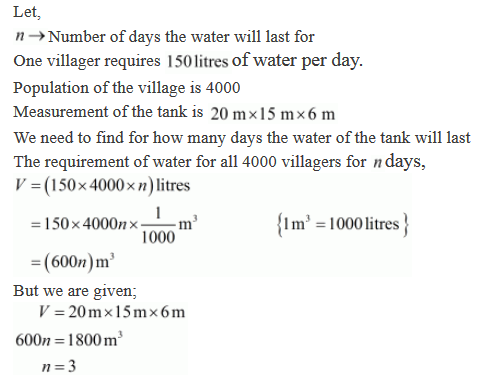26. A child playing with building blocks, which are of the shape of the cubes, has built a structure as shown in Fig. 18.12 If the edge of each cube is 3 cm, find the volume of the structure built by the child.Solution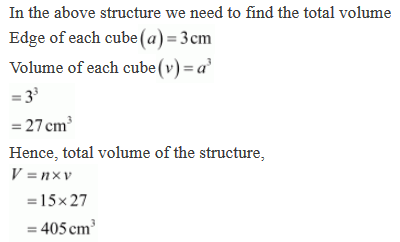27. A godown measure 40 m × 25 m × 10 m. Find the maximum number of wooden crates each measuring 1.5 m × 1.25 m × 0.5 m that can be stored in the godown.

Solution28. A wall of length 10 m was to be built across an open ground. The height of the wall is 4 m and thickness of the wall is 24 cm. If this wall is to be built up with bricks whose dimensions are 24 cm × 12cm × 8cm, how many bricks would be required.

Solution